#Function Repository Resource:

# ImplicitD (1.0.0)current version: 3.0.1 »

Compute the derivative y' as a function of x from an implicit equation in those variables

Contributed by: Wolfram|Alpha Math Team
 ResourceFunction["ImplicitD"][eqn,D[y,x]] returns the implicit derivative ∂y/∂x as determined from equation eqn. ResourceFunction["ImplicitD"][eqn,D[y,x],pt] returns the implicit derivative ∂y/∂x at the point pt.

## Details and Options

With "EliminateDependentVariables"True, ResourceFunction["ImplicitD"] will attempt to eliminate the dependent variable from the output expression by substituting its original value from the input equation.
ResourceFunction["ImplicitD"] has the attribute HoldRest, so that the D[y,x] argument does not autoevaluate to 0.

## Examples

### Basic Examples (2)

Compute the implicit derivative ∂y/∂x on the unit circle:

 In:=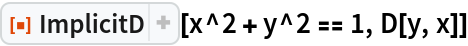Out=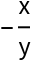Compute the implicit derivative at a specific point:

 In:=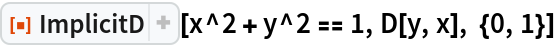Out=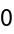### Scope (1)

ImplicitD treats non-input variables as constants:

 In:=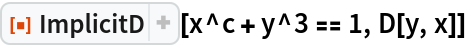Out=In:=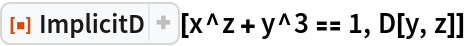Out=### Options (1)

With "EliminateDependentVariables"True, ImplicitD will attempt to eliminate the dependent variable from the output expression by substituting its original value from the input equation:

 In:=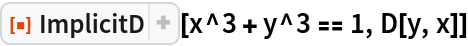Out=In:=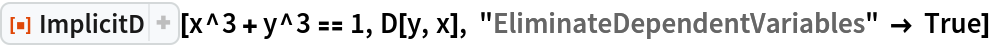Out=### Possible Issues (1)

If the derivative of a variable that does not appear in the expression is requested, ImplicitD will return unevaluated:

 In:=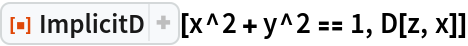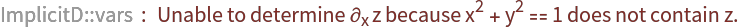Out=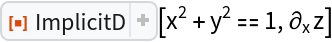## Publisher

Wolfram|Alpha Math Team

## Version History

• 3.0.1 – 27 March 2023
• 3.0.0 – 23 March 2023
• 2.0.0 – 23 March 2023
• 1.0.0 – 10 April 2020